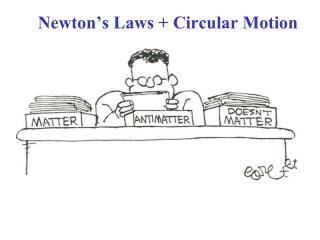Download PresentationNewton’s Laws + Circular Motion# Newton’s Laws + Circular Motion - PowerPoint PPT Presentation

Download Presentation##### Newton’s Laws + Circular Motion

Download Policy: Content on the Website is provided to you AS IS for your information and personal use and may not be sold / licensed / shared on other websites without getting consent from its author. While downloading, if for some reason you are not able to download a presentation, the publisher may have deleted the file from their server.

- - - - - - - - - - - - - - - - - - - - - - - - - - - E N D - - - - - - - - - - - - - - - - - - - - - - - - - - -
##### Presentation Transcript

1. Newton’s Laws + Circular Motion

2. Sect. 5-2: Uniform Circular Motion; Dynamics A particle moving in uniform circular motion at radius r, speed v = constant: • Centripetal acceleration: aR = (v2/r) , aR v always!! aR is radially inward always! • Newton’s 1st Law: There must be a force acting! • Newton’s 2nd Law: ∑F = ma = maR = m(v2/r)(magnitude) Direction: The total force must be radially inward always! For an object to be in uniform circular motion,there must be a net force acting on it. We know the acceleration, so we can immediately write the force:

3. A force is required to keep an object moving in a circle.If the speed is constant, the force is directed toward the center of the circle. The direction of the force is continually changing so that it is always pointed toward the center of the circle. Example:A ball twirled on the end of a string. In that case, the force is the tension in the string.

4. A particle moving in uniform circular motion, radius r(speed v = constant) • The acceleration: aR = (v2/r), aR v always!! aR is radially inward always! • Newton’s 1st Law:There mustbe a force acting! • Newton’s 2nd Law:∑F = ma = maR= m(v2/r) The total force ∑Fmust be radially inward always!  The force which enters N’s 2nd Law  Centripetal Force (Center directed force) • NOTa new kind of force! Could be string tension, gravity, etc. It’s the right side of ∑F = ma, not the left side! (It’s the form of “ma”, for circular motion!)

5. Centripetal Force You can understand that the centripetal force must be inward by thinking about the ball on a string. Strings only pull; they never push. MISCONCEPTION!! The force on the ball is NEVER Outward (“Centrifugal”).It is ALWAYSinward(Centripetal) !!

6. An outward(“centrifugal”) force ON THE BALL is NOT a valid concept! The string tension force ON THE BALL is inward(centripetal). F The string tension forceFon the ball isINWARDtoward the center of the circle!

7. What happens if the cord on the ball is broken or released? For the ball to move in a circle, theremust be an inward(Centripetal)forcepointed towards the circle center so that the natural tendency of the object to move in a straight line(Newton’s 1st Law!)will be overcome. If the centripetal force goes to zero, the ball will fly off in a direction tangent to the circle(Newton’s 1st Lawagain!) There isno centrifugal forcepointing outward on the ball!

8. Example Sparks fly in straight line tangentially from the edge of a rotating grinding wheel

9. Example 5-3 (Estimate) Estimate the force a person must exert on a string attached to a 0.15 kg ball to make the ball revolve in a horizontal circle of radius 0.6 m. The ball makes 2 rev/s. m = 0.15 kg, r = 0.6 m, f = 2 rev/s  T = 0.5 s Assumption:Circular path is  in horizontal plane, so φ 0  cos(φ)  1 Newton’s 2nd Law: ∑F = ma  FTx = max= maR = m(v2/r) v = (2πr/T) = 7.54 m/s So, the tension is (approximately) FTx  14 N

10. Example 5-4: Revolving ball (vertical circle) A ball, mass m = 0.15 kg on the end of a (massless) cord of length r = 1.1 m cord is swung in a verticalcircle. Calculate: a. The minimum speed the ball must have at the top of its arc so that the ball continues moving in a circle. b. The tension in the cord at the bottom of the arc, assuming that there the ball is moving at twice the speed found in part a. Hint:The minimum speed at the top will happen for the minimum tensionFT1 Note:Here, string tension & gravity are acting together (both enter Newton’s 2nd Law!) to produce centripetal acceleration.

11. Problem 7 r = 0.72 m, v = 4 m/s, m = 0.3 kg Use: ∑F = maR • At the top of the circle: Vertical forces: (down is positive!) FT1 + mg = m(v2/r) FT1 = 3.73 N • At the bottom of the circle: Vertical forces: (up is positive!) FT2 - mg = m(v2/r) FT2 = 9.61 N

12. Example: Exercise C, p. 111 A Ferris wheel rider moves in a vertical circle of radius r at constant speed v. Is the normal force FN1 that the seat exerts on the rider at the top of the wheel a. less than, b. more than, or c. equal to the normal force FN2 that the seat exerts on the rider at the bottom of the wheel? Use Newton’s 2nd Law:∑F = maR at top & bottom, solve for normal force & compare. 1 2

13. Conceptual Example 5-5 A tether ball is hit so that it revolves around a pole in a circle of radius r at constant speed v. In what direction is the acceleration? What force causes it? Newton’s 2nd Law:∑F = ma x: ∑Fx = max  FTx =maR = m(v2/r) y: ∑Fy = may = 0  FTy -mg = 0, FTy =mg